# The meaning of lim x → a f ( x ) = L .### Single Variable Calculus: Concepts...

4th Edition
James Stewart
Publisher: Cengage Learning
ISBN: 9781337687805### Single Variable Calculus: Concepts...

4th Edition
James Stewart
Publisher: Cengage Learning
ISBN: 9781337687805

#### Solutions

Chapter 2, Problem 1RCC

(a)

To determine

## To explain: The meaning of limx→af(x)=L.

Expert Solution

### Explanation of Solution

Result used:

Definition of limit:

Let f(x) be a function is defined when x approaches to p then limxpf(x)=L, if for every number ε>0 there is some δ>0  such that |f(x)L|<ε whenever 0<|xp|<δ.

Graph: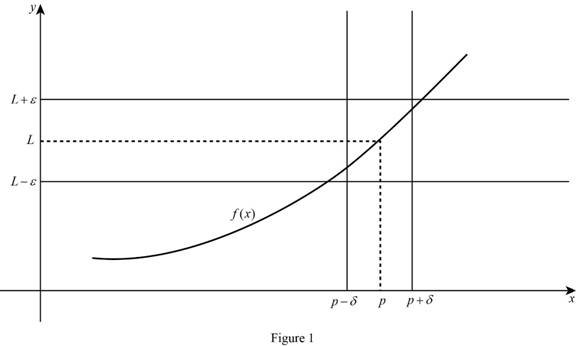Calculation:

The limit of the function limxaf(x)=L means the limit of f(x) equal to L when x approaches to a, if x is closer and closer to a from the both sides then the value of f(x) also closer and closer to L.

In the limit definition xa this means finding the limit of f(x) when x approaches to a, there no need to consider x=a.

There are three cases for define limxaf(x)=L.

Case (1):

The limit of the function limxaf(x)=L, if x approaches to a then the value of f(x) are closer to L and f(a) is L.

Graph: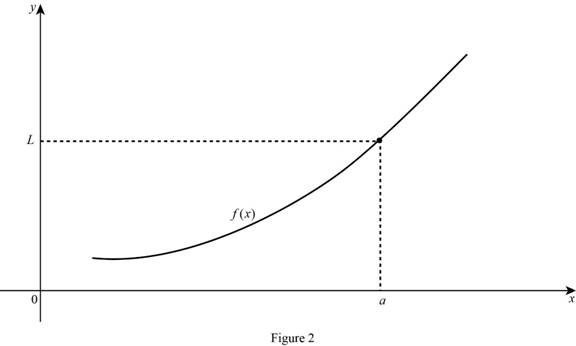Case (2):

The limit of the function limxaf(x)=L, if x approaches to a then the value of f(x) are closer to L and f(a) is undefined.

Case (3):

The limit of the function limxaf(x)=L, if x approaches to a then the value of f(x) are closer to other than L.

Graph: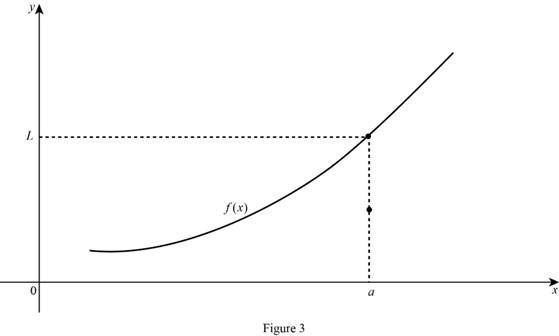(b)

To determine

Expert Solution

### Explanation of Solution

Result used:

Definition of limit:

Let f(x) be a function is defined when x approaches to p then limxpf(x)=L, if for every number ε>0 there is some δ>0  such that |f(x)L|<ε whenever 0<|xp|<δ.

Calculation:

limxa+f(x)=L means the limit of f(x) equal to L when x approaches to a from the right, if x is closer and closer to a from the right and remains greater than a then the value of f(x) also closer and closer to L.

Graph: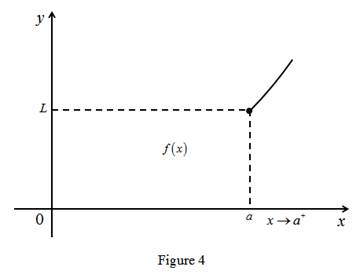(c)

To determine

Expert Solution

### Explanation of Solution

Result used:

Definition of limit:

Let f(x) be a function is defined when x approaches to p then limxpf(x)=L, if for every number ε>0 there is some δ>0  such that |f(x)L|<ε whenever 0<|xp|<δ.

Calculation:

The limit of the function limxaf(x)=L means the limit of f(x) equal to L when x approaches to a from the left, if x is closer and closer to a from the left and remains less than a then the value of f(x) also closer and closer to L.

Graph: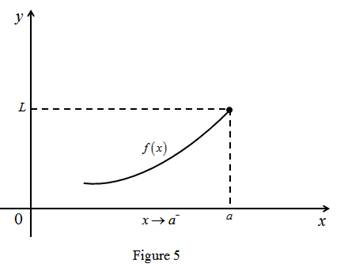(d)

To determine

Expert Solution

### Explanation of Solution

Result used:

Definition of limit:

Let f(x) be a function is defined when x approaches to p then limxpf(x)=L, if for every number ε>0 there is some δ>0  such that |f(x)L|<ε whenever 0<|xp|<δ.

Calculation:

The limit of the function limxaf(x)= means the limit of f(x) is larger value when x approaches to a from the both sides. That is any M>0, f(x)>M for some x-value is sufficiently close to a.

Graph: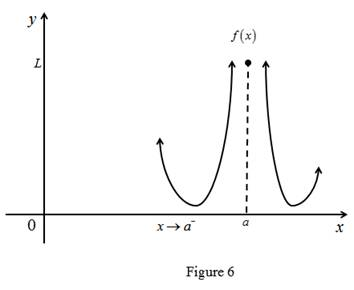(e)

To determine

Expert Solution

### Explanation of Solution

Result used:

Definition of limit:

Let f(x) be a function is defined when x approaches to p then limxpf(x)=L, if for every number ε>0 there is some δ>0  such that |f(x)L|<ε whenever 0<|xp|<δ.

Calculation:

The limit of the function limxf(x)=L means the limit of f(x) is L when x approaches to larger value the graph get closer and closer to the line y=L.

Graph: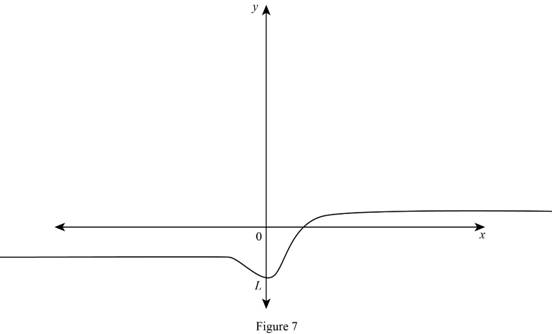### Want to see more full solutions like this?

Subscribe now to access step-by-step solutions to millions of textbook problems written by subject matter experts!

### Have a homework question?

Subscribe to bartleby learn! Ask subject matter experts 30 homework questions each month. Plus, you’ll have access to millions of step-by-step textbook answers!